Courses

# Test: Differential Equations & Multiple Integrals- 1

## 20 Questions MCQ Test GATE Civil Engineering (CE) 2022 Mock Test Series | Test: Differential Equations & Multiple Integrals- 1

Description
This mock test of Test: Differential Equations & Multiple Integrals- 1 for GATE helps you for every GATE entrance exam. This contains 20 Multiple Choice Questions for GATE Test: Differential Equations & Multiple Integrals- 1 (mcq) to study with solutions a complete question bank. The solved questions answers in this Test: Differential Equations & Multiple Integrals- 1 quiz give you a good mix of easy questions and tough questions. GATE students definitely take this Test: Differential Equations & Multiple Integrals- 1 exercise for a better result in the exam. You can find other Test: Differential Equations & Multiple Integrals- 1 extra questions, long questions & short questions for GATE on EduRev as well by searching above.
QUESTION: 1

### A triangle ABC consists of vertex points A (0,0) B(1,0) and C(0,1). The value of the integral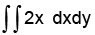over the triangle is

Solution:

The equation of the line  AB is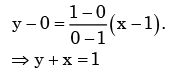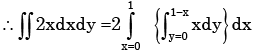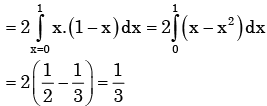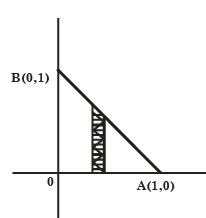QUESTION: 2

### The area enclosed between the parabala y = x2 and the straight line     y = x is

Solution: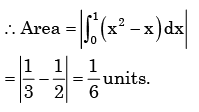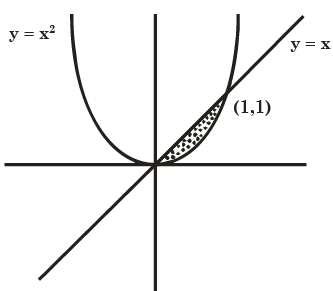QUESTION: 3

### Changing the order of the integration in the double integral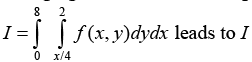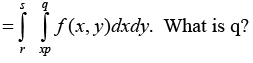Solution:

When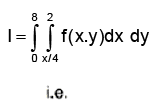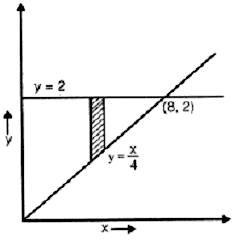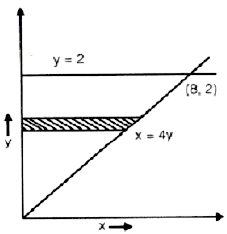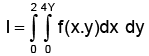QUESTION: 4

The right circular cone of largest volume that can be enclosed by a sphere of 1 m radius has a height of

Solution: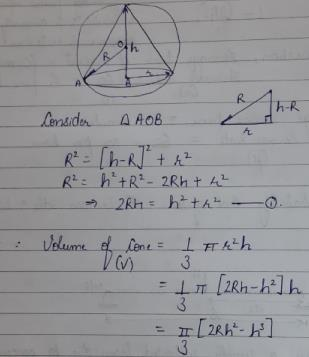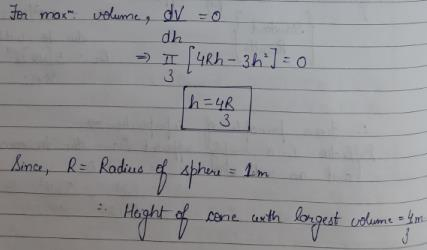QUESTION: 5

the parabolic arc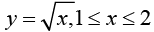is revolved around the x-axis. The volume of

Solution:

Differential volume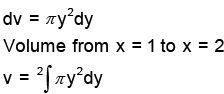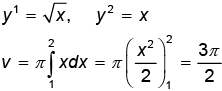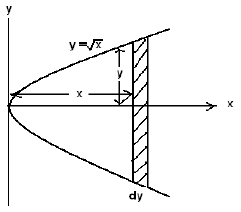QUESTION: 6

What is the area common to the circles r = a and r = 2a cos θ?

Solution:

Area common to circles r = a

And r = 2a cos θ is 1.228 a2

QUESTION: 7

The expression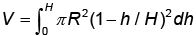for the volume of a cone is equal to

Solution:

Choices (a) and (b)  be correct because

for the volume of a cone is equal to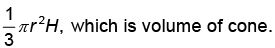QUESTION: 8

f ( x,y ) is a continuous defined over ( x,y ) ∈ [0,1]× [0,1] . Given two constrains, x > y 2 and y > x 2 , the volume under f ( x,y ) is

Solution: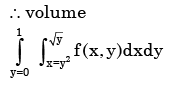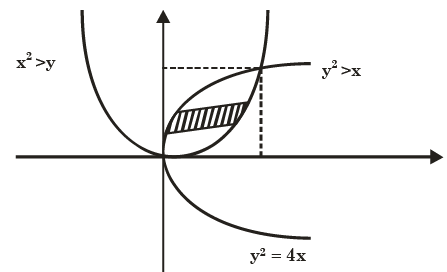QUESTION: 9

The following differential equation has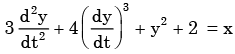Solution: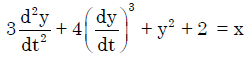Order of highest derivative = 2
Hence, most appropriate answer is (b)

QUESTION: 10

A solution of the following differential equation is given by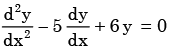Solution:

Let y = mx be the trial soln of the given differental equation
∴The corrosponding auxiliary equation is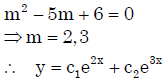QUESTION: 11

For the differential equation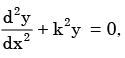the boundary conditions are

(i)  y = 0 for x = 0, and

(ii) y = 0 for x = a

The form of non-zero solutions of y (where m varies over all integers) are

Solution:

Here y = c1 coskx + c2 sinkx ........... (1) be the solution of the given differential equation.
Now use boundary conditions
For x = 0,y = 0 gives c1 = 0. Equation − (1) becomes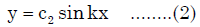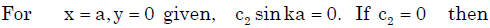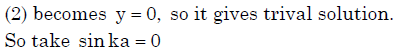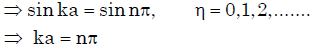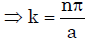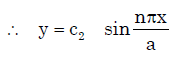be the solution, n 0,1,2,3.......

QUESTION: 12

Which of the following is a solution to the differential equation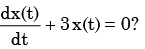Solution:

Hints : m + 3 = 0 ⇒m = −3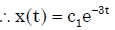QUESTION: 13

For the differential equation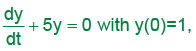the general solution is

Solution: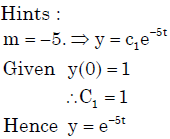QUESTION: 14

The solution of the differential equation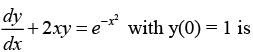Solution:

It is a linear diff. equation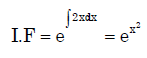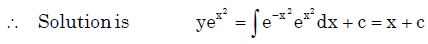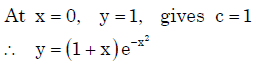QUESTION: 15

The solution of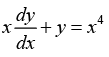with the condition y(1)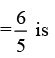Solution: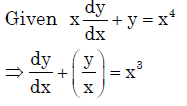Which is 1st order linear differential equation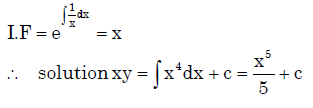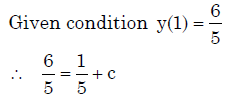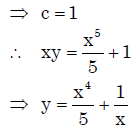QUESTION: 16

A technique by which problems in analysis, in particular differential equations, are transformed into algebraic problems is called

Solution:
QUESTION: 17

The general solution of (x2 D2 – xD), y= 0 is :

Solution:
QUESTION: 18

For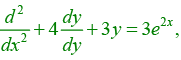the particular integrals is

Solution: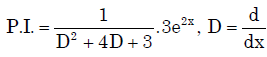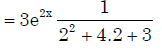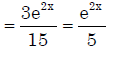QUESTION: 19

It is given that y" + 2y' + y = 0, y(0) = 0, y(1)=0. What is y (0.5)?

Solution:

Auxiliary equation is m2 + 2m +1 = 0⇒m = −1,−1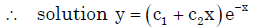Using boundary condition y(0) = 0 and y(1) = 0
we get y = 0

QUESTION: 20

The partial differential equation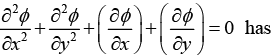Solution:

The order is the highest numbered derivative in the equation (which is 2 here), while the degree is the highest power to which a derivative is raised (which is 1 here).